ConceptDraw
DIAGRAM 16

# Fault Tree Analysis Example

Designing the Fault Tree Analysis (FTA) Diagrams first of all it is important to have a powerful software. ConceptDraw DIAGRAM diagramming and vector drawing software extended with Fault Tree Analysis Diagrams Solution from the Industrial Engineering Area is exactly what you need. It is also convenient to have professional looking Fault Tree Analysis example before the eyes, and it is excellent that this solution includes large collection of such examples.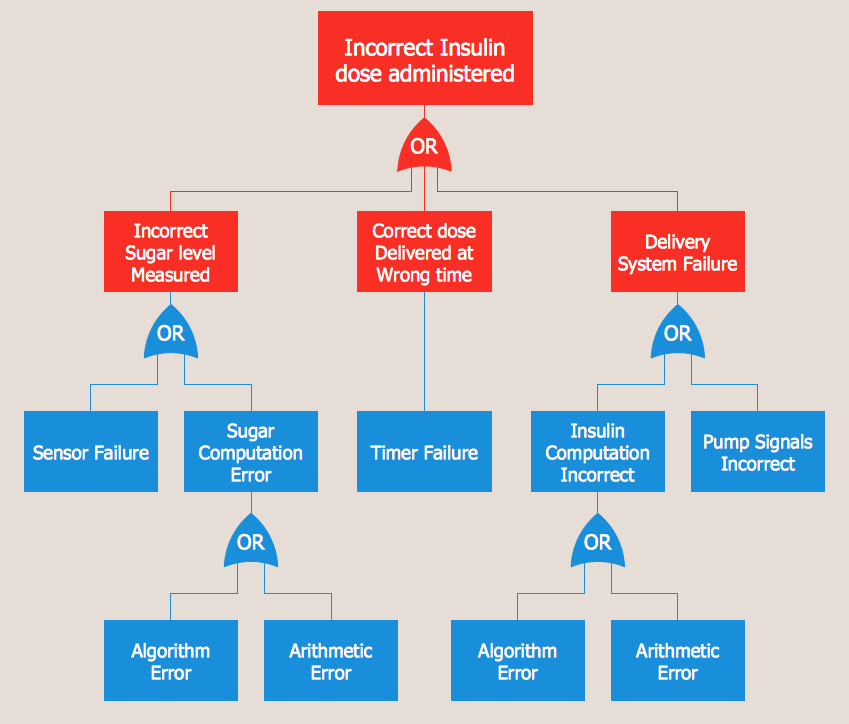Example 1. Fault Tree Analysis Example

Fault Tree Analysis Diagrams Solution is rich for predesigned samples, templates, and ready-to-use vector elements developed and grouped in Fault Tree Analysis Diagram library.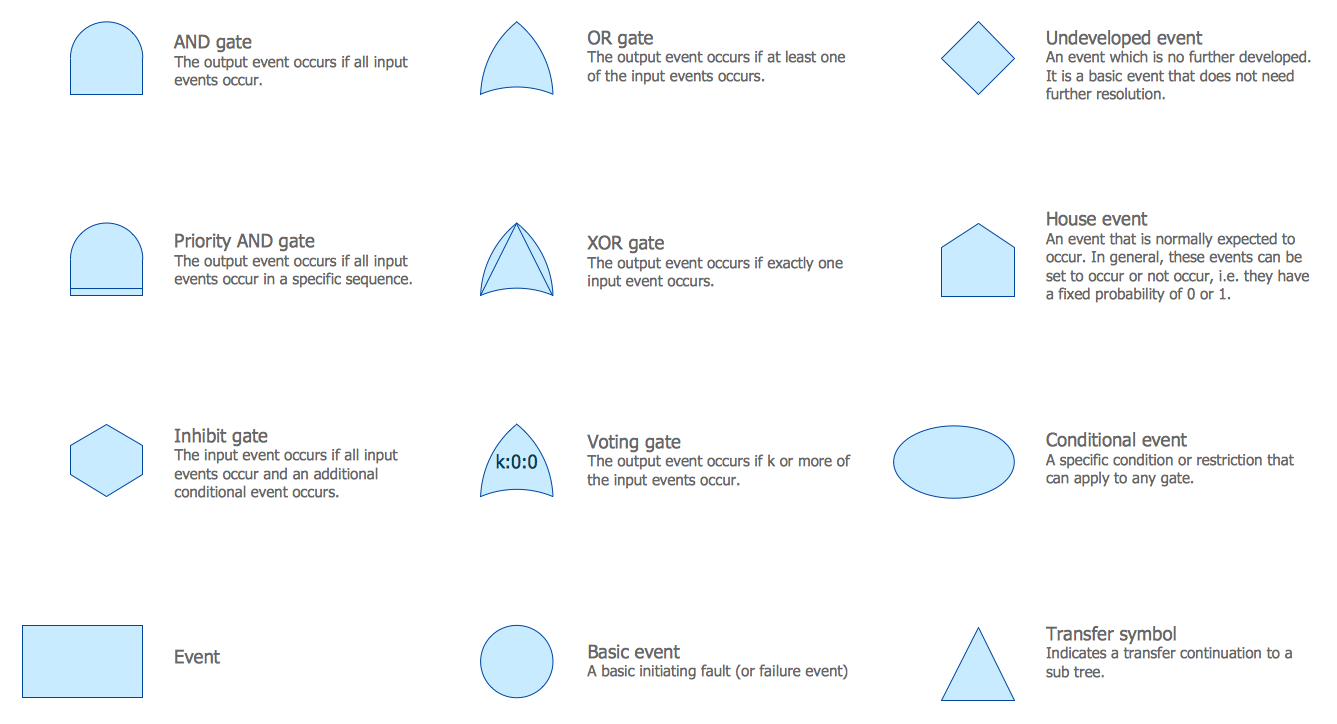Example 2. Fault Tree Analysis Diagram Library Design Elements

Variety of samples is available from ConceptDraw STORE. Any Fault Tree Analysis example or template included in Fault Tree Analysis Diagrams Solution can be used as the base for quickly design your own FTA Diagram.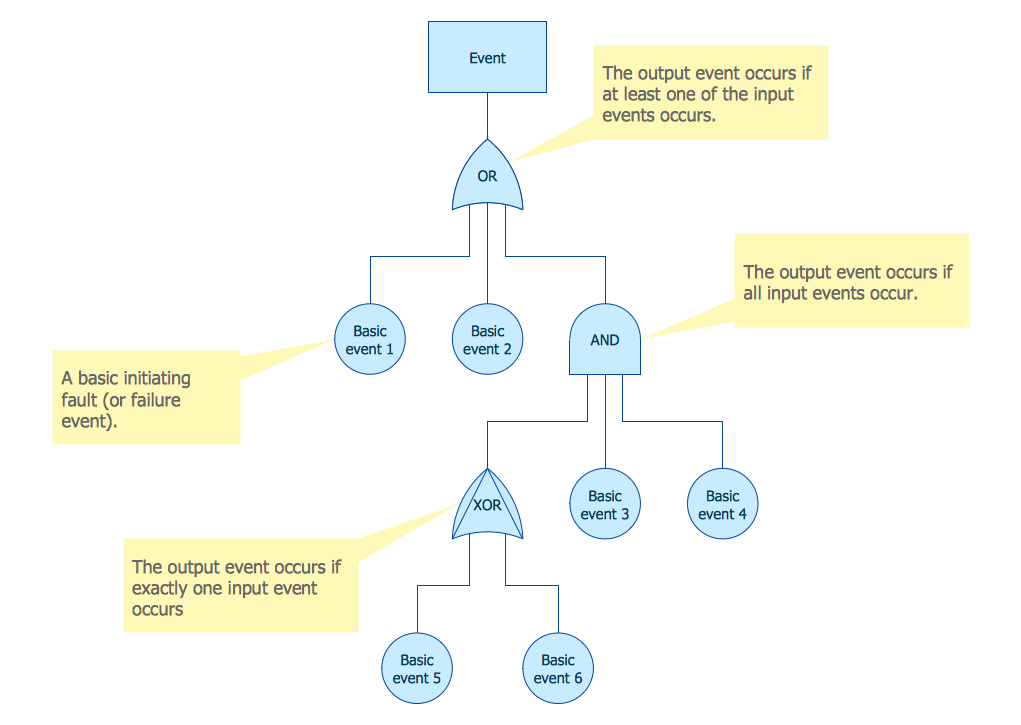Example 3. Fault Tree Analysis Diagram Template

The Fault Tree Analysis example and template you see on this page were created in ConceptDraw DIAGRAM using the Fault Tree Analysis Diagrams Solution. You can find them in Fault Tree Analysis Diagrams section of ConceptDraw STORE.

Use the Fault Tree Analysis Diagrams Solution for ConceptDraw DIAGRAM software to create your own professional looking Fault Tree Analysis Diagrams quick, easy and effective.

All source documents are vector graphic documents. They are available for reviewing, modifying, or converting to a variety of formats (PDF file, MS PowerPoint, MS Visio, and many other graphic formats) from the ConceptDraw STORE. The Fault Tree Analysis Diagrams Solution is available for all ConceptDraw DIAGRAM or later users.

### Fault Tree Analysis Software →

ConceptDraw DIAGRAM extended with Fault Tree Analysis Diagrams Solution from the Industrial Engineering Area of ConceptDraw Solution Park is the best Fault Tree Analysis Software. First of all, Fault Tree Analysis Diagrams Solution provides a set of samples which are the good examples of easy drawing professional looking Fault Tree Analysis Diagrams.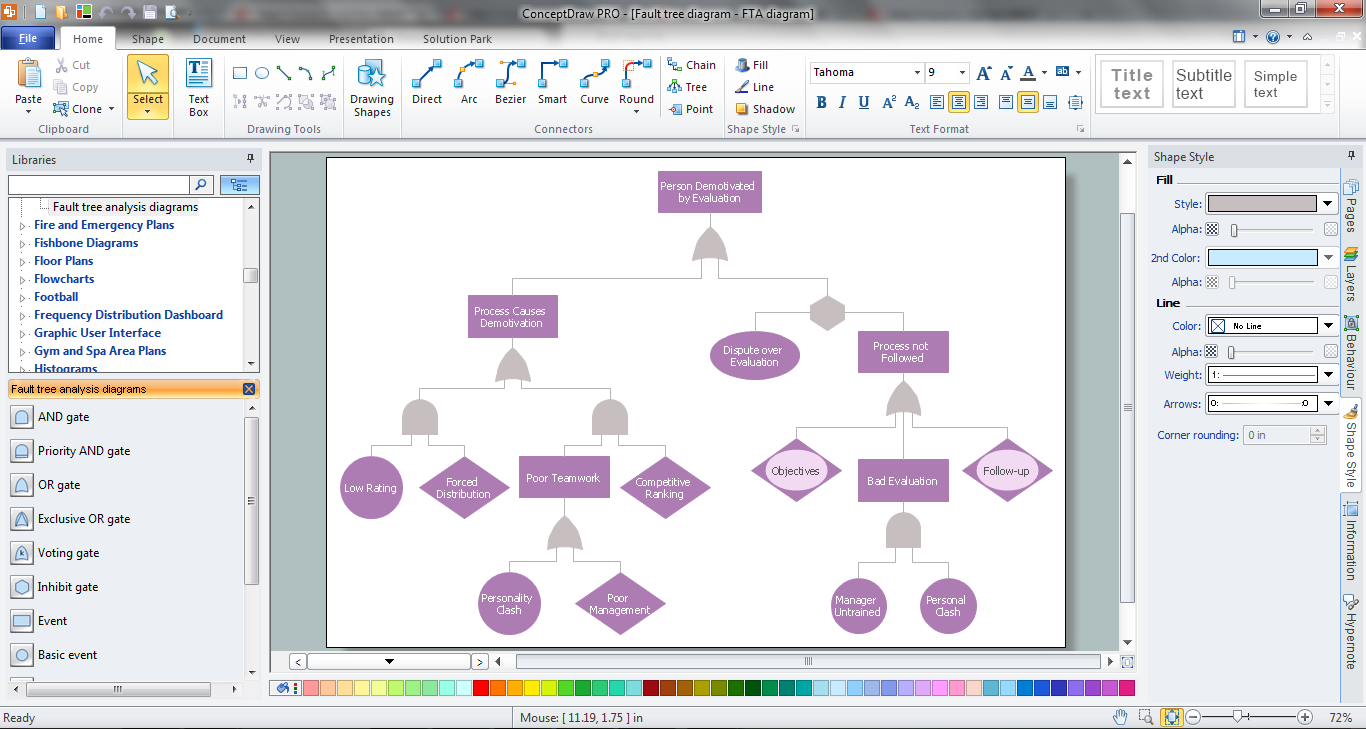Picture: Fault Tree Analysis Software
Related Solution:

### Fault Tree Diagram →

ConceptDraw DIAGRAM diagramming and vector drawing software offers the Fault Tree Analysis Diagrams Solution from the Industrial Engineering Area of ConceptDraw Solution Park for quick and easy creating the Fault Tree Diagram of any degree of detailing.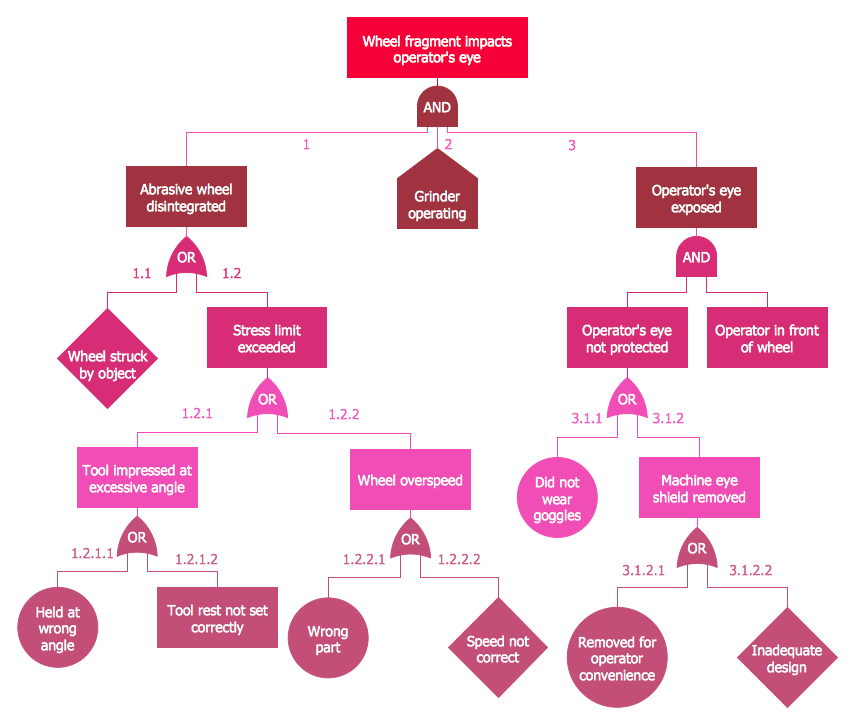Picture: Fault Tree Diagram
Related Solution:

### Network Topologies →

When describing any computer network, we imagine a set of devices and nodes, arranged in some way. Talking about network structures, we should distinguish physical and logical network topologies, as physical topology is about devices location and logical topology illustrates data flow. In the same time, they do not have to match, and some devices, such as repeaters, may have a physical star layout, but a bus logical topology. There are two main types of computer network topologies: Physical topology that show the physical organization of a network - equipment and types of connections. Star network topology involves a set of devices that is connected to a single hub (router). Ring network topology means that, devices connected according this topology have two connections, connecting with nearby devices to make a loop. Bus network topology is the topology presented at the current diagram. It is similar to a ring topology. The difference is that data moves up and down a linear connection, copying itself where network equipment works as bus-stations along the way. This network topology can be used for small network, or when adding an extra device into a network.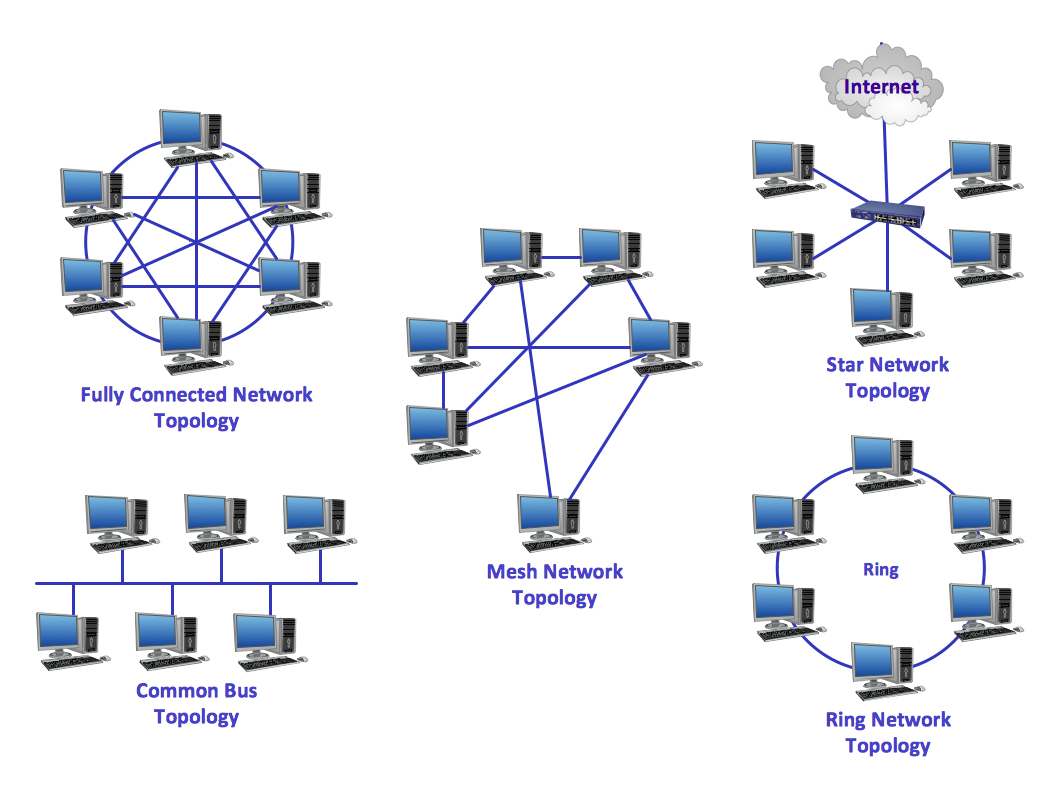Picture: Network Topologies
Related Solution:
ConceptDraw
DIAGRAM 16